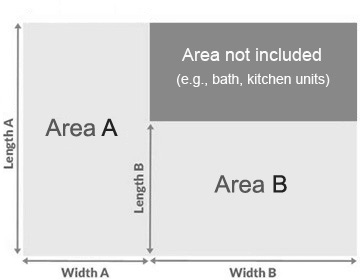# Room Size Calculator - Calculate the Size of the Room

Dividing the room into a particular number of separate sections is an effective way to calculate the room's precise size when it has an irregular shape. For example, if your room has a rectangular shape, you should write down only its width and depth in the calculator, and specify the units of measurement. The calculator will provide you with the floor area's value, which can be calculated in distinct units of measurement, such as imperial and metric. However, in most cases, rooms do not have a perfect rectangular shape. That's why we recommend dividing the room into rectangular sections, as mentioned above, and then calculating the sizes of each rectangle separately. Finally, add up all the values to get the total area. To start calculating, enter all the dimensions and choose the correct units of measurement.Room Size Calculator

Select Feet or Meters

m.

m.

m.

m.

ft. in.

ft. in.

ft. in.

ft. in.

### Results:

Total Area: 0 sq. ft.
Allowing 10% Wastage: 0 sq. ft.